WHCSRL 技术网

# C语言函数、函数声明、定义、递归、汉诺塔问题、青蛙跳台阶问题

1. 库函数
2. 自定义函数

## 2.1 库函数：

1. 我们知道在我们学习C语言编程的时候，总是在一个代码编写完成之后迫不及待的想知道结果，想把这个结果打印到我们的屏幕上看看。这个时候我们会频繁的使用一个功能：将信息按照一定的格式打印到屏幕上（printf）。
2. 在编程的过程中我们会频繁的做一些字符串的拷贝工作（strcpy）。
3. 在编程是我们也计算，总是会计算n的k次方这样的运算（pow）。

## 2.2自定义函数

``````ret_type fun_name(para1, * )
{
statement;//语句项
}
ret_type 返回类型
fun_name 函数名
para1    函数参数
```1234567```

``````#include<stdio.h>
int get_max(int x, int y)
{
return(x > y ? x : y);
}
int main()
{
int a = 0;
int b = 0;
scanf("%%%%d%%%%d", &a, &b);

//求最大值
int max = get_max(a, b);
printf("max = %%%%d
", max);
return 0;
}
```12345678910111213141516```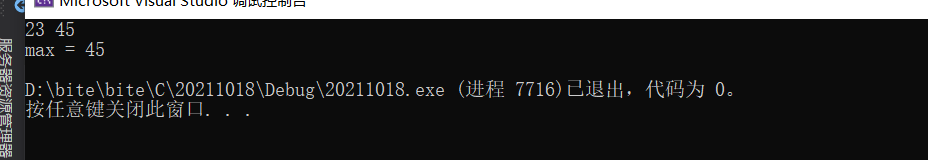``````#include<stdio.h>
void Swap(int x, int y)
{
int z = 0;
z = x;
x = y;
y = z;
}
int main()
{
int a = 10;
int b = 20;

printf("交换前：a=%%%%d b=%%%%d
", a, b);
//函数
Swap(a, b);
printf("交换后：a=%%%%d b=%%%%d
", a, b);
return 0;
}
```12345678910111213141516171819```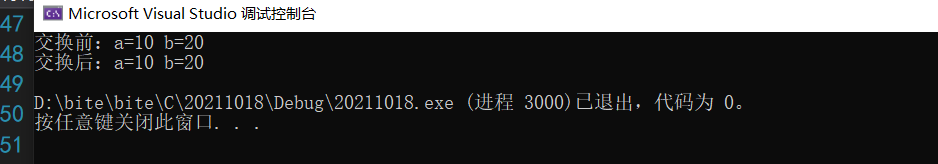1. 实参a和b传给形参x和y的时候，形参将是实参的临时拷贝
2. 改变形参变量x和y，不会影响实参a和b

`````` #include<stdio.h>
void Swap(int* px, int* py)
{
int z = 0;
z = *px;
*px = *py;
*py = z;
}

int main()
{
int a = 10;
int b = 20;
printf("交换前：a=%%%%d b=%%%%d
", a, b);
//函数
Swap(&a, &b);
printf("交换后：a=%%%%d b=%%%%d
", a, b);
return 0;
}
```12345678910111213141516171819```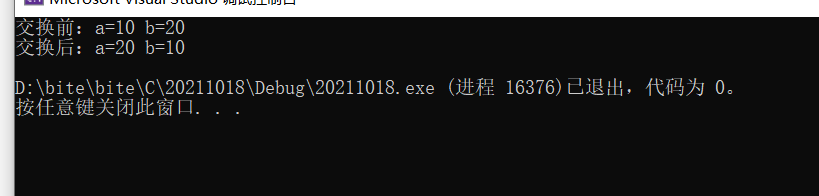## 3.1 实际参数（实参）：

1. 真实传给函数的参数，叫实参。
2. 实参可以是：常量、变量、表达式、函数等。
3. 无论实参是何种类型的量，在进行函数调用时，它们都必须有确定的值，以便把这些值传送给形参。

## 3.2形式参数（形参）：

1. 形式参数是指函数名后括号中的变量，因为形式参数只有在函数被调用的过程中才实例（分配内存单元），所以叫形式参数。形式参数当函数调用完成之后就自动销毁了。因此形式参数只在函数中有效。
2. 上面Swap1和Swap2函数中的参数 x，y，px，py 都是形式参数。在main函数中传给Swap1的num1，num2和传给Swap2函数的&num1，&num2是实际参数。

## 4.3 练习

1. 写一个函数可以判断一个数是不是素数。
``````//1. 写一个函数可以判断一个数是不是素数。
#include<stdio.h>
#include<math.h>
int is_prime(int n)
{
//试除法
//2--n-1
//2--sqrt(n)
int j = 0;
for (j = 2; j <= sqrt(n); j++)
{
if (n %%%% j == 0)
return 0;
}
return 1;
}
int main()
{
//100--200之间的素数
int i = 0;
for (i = 100; i <= 200; i++)
{
//判断是否为素数
if (is_prime(i) == 1)
{
printf("%%%%d ", i);
}
}
return 0;
}
```123456789101112131415161718192021222324252627282930```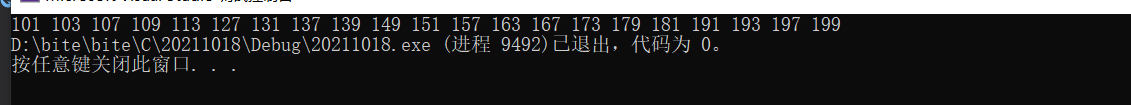2. 写一个函数判断一年是不是闰年。

``````#include<stdio.h>
int is_leap_year(int year)
{
if ((year %%%% 100 != 0 && year %%%% 4 == 0) || (year %%%% 400 == 0))
{
return 1;
}
else
{
return 0;
}
}

int main()
{
//1000--2000之间的闰年
int y = 0;
for (y = 1000; y <= 2000; y++)
{
//判断是否为闰年
//如果是闰年，返回1
//不是闰年，返回0
if (is_leap_year(y) == 1)
{
printf("%%%%d ", y);
}
}
return 0;
}
```1234567891011121314151617181920212223242526272829```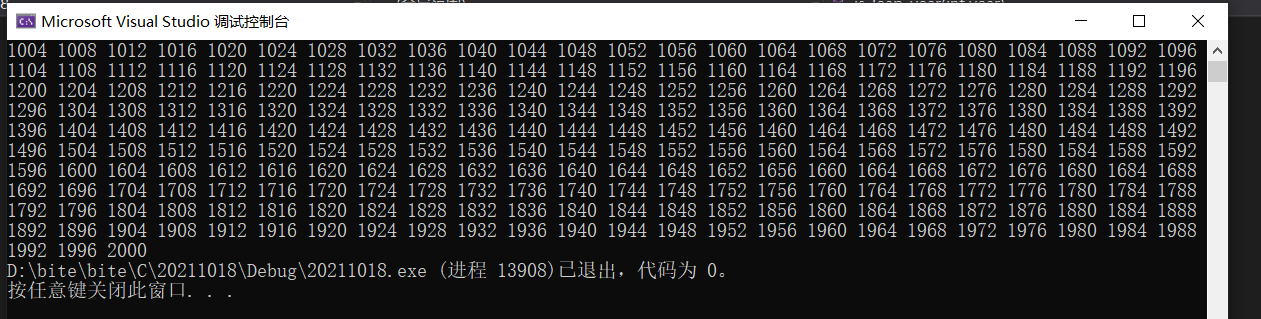3. 写一个函数，实现一个整形有序数组的二分查找。

``````//3. 写一个函数，实现一个整形有序数组的二分查找。
#include<stdio.h>
int binary_search(int arr[],int n, int size)
{
int left = 0;
int right = size - 1;
while (left <= right)
{
int mid = (left + right) / 2;
if (n < arr[mid])
right = mid - 1;
else if (n > arr[mid])
left = mid + 1;
else
return mid;
}
return -1;
}

int main()
{
int arr = { 1,2,3,4,5,6,7,8,9,10 };
int k = 7;
//计算数组元素的个数
int sz = sizeof(arr) / sizeof(int);

//找到了返回下标
//找不到返回-1
int ret = binary_search(arr, k, sz);//测试驱动开发
if (ret == -1)
printf("找不到
");
else
printf("找到了,下标是：%%%%d
",ret);
return 0;
}
```1234567891011121314151617181920212223242526272829303132333435```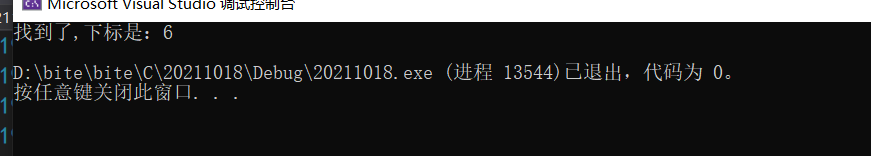``````#include<stdio.h>
int binary_search(int arr[], int n, int left,int right)
{
while (left <= right)
{
int mid = (left + right) / 2;
if (n < arr[mid])
right = mid - 1;
else if (n > arr[mid])
left = mid + 1;
else
return mid;
}
return -1;
}

int main()
{
int arr = { 1,2,3,4,5,6,7,8,9,10 };
int k = 3;
//计算数组元素的个数
int sz = sizeof(arr) / sizeof(int);

//找到了返回下标
//找不到返回-1
int ret = binary_search(arr, k, 0, 9);//测试驱动开发
if (ret == -1)
printf("找不到
");
else
printf("找到了,下标是：%%%%d
", ret);
return 0;
}
```123456789101112131415161718192021222324252627282930313233```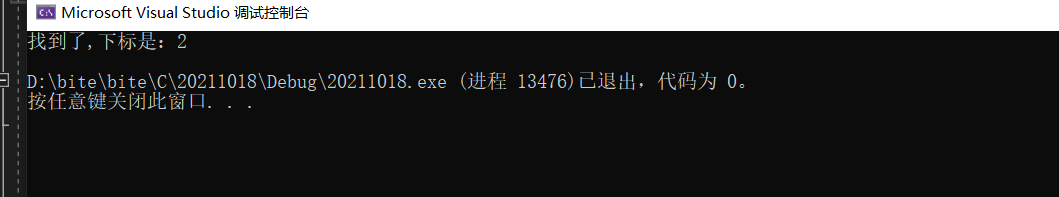1. 写一个函数，每调用一次这个函数，就会将num的值增加1。
题目的意思就是函数可以改变变量num的值
``````#include<stdio.h>
{
*p = *p + 1;
}
int main()
{
int num = 0;
printf("%%%%d
", num);//1
printf("%%%%d
", num);//2
printf("%%%%d
", num);//3
return 0;
}
```12345678910111213141516```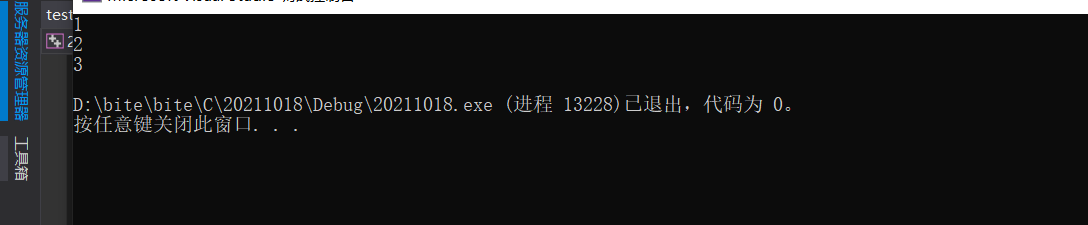``````#include<stdio.h>
{
return n + 1;
}
int main()
{
int num = 0;
printf("%%%%d
", num);//1
printf("%%%%d
", num);//2
printf("%%%%d
", num);//3
return 0;
}
```12345678910111213141516```

## 5.1嵌套调用

``````#include <stdio.h>
void new_line()
{
printf("hehe
");
}
void three_line()
{
int i = 0;
for(i=0; i<3; i++)
{
new_line();
}
}
int main()
{
three_line();
return 0;
}
```123456789101112131415161718```

## 5.2 链式访问

``````#include <stdio.h>
#include <string.h>
int main()
{
char arr = "hello";
int ret = strlen(strcat(arr, "bit"));//这里介绍一下strlen函数
printf("%%%%d
", ret);
return 0;
}
```123456789```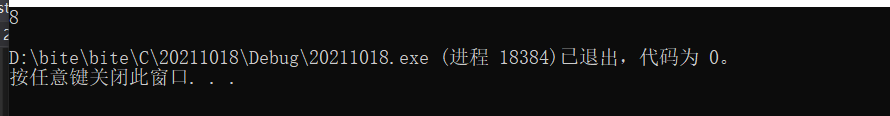``````#include <stdio.h>
int main()
{
printf("%%%%d", printf("%%%%d", printf("%%%%d", 43)));
//结果是啥？
//注：printf函数的返回值是打印在屏幕上字符的个数
return 0;
}
```12345678```

printf返回在屏幕打印字符的个数,

``````printf("%%%%d", printf("%%%%d", printf("%%%%d", 43)));
printf("%%%%d", printf("%%%%d", 2));
printf("%%%%d", 1)
```123```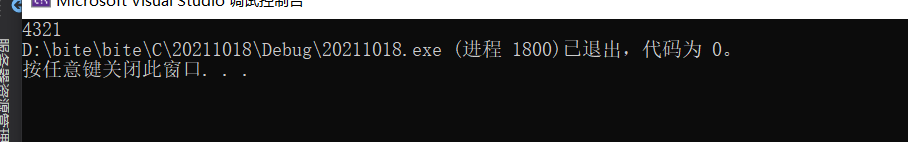## 6.1 函数声明：

1. 告诉编译器有一个函数叫什么，参数是什么，返回类型是什么。但是具体是不是存在，函数声明决定不了。
2. 函数的声明一般出现在函数的使用之前。要满足先声明后使用。
3. 函数的声明一般要放在头文件中的。

## 6.2 函数定义：

``````//test.h的内容
//放置函数的声明
#ifndef __TEST_H__
#define __TEST_H__
//函数的声明
int Add(int x, int y);
#endif //__TEST_H__
```1234567```
``````//test.c的内容
//放置函数的实现
#include "test.h"
int Add(int x, int y)
{
return x+y;
}
```12345678```

## 7.2 递归的两个必要条件

1. 存在限制条件，当满足这个限制条件的时候，递归便不再继续。
2. 每次递归调用之后越来越接近这个限制条件。

### 7.2.1 练习1：

``````#include <stdio.h>
void print(int n) {
if(n>9)
{
print(n/10);
}
printf("%%%%d ", n%%%%10);
}
int main()
{
int num = 1234;
print(num);
return 0;
}
```1234567891011121314```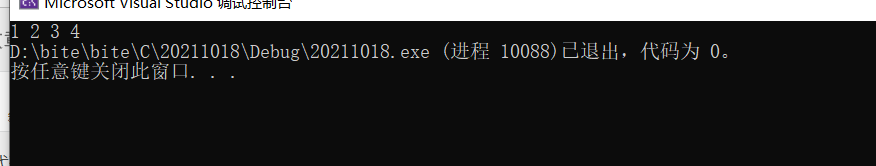### 7.2.2 练习2:

``````//递归求字符串长度
#include<stdio.h>
int my_strlen(char* s)
{
if (*s != '')
{
return 1 + my_strlen(s + 1);
}
else
{
return 0;
}
}
int main() {
//求字符串长度
char arr = "abcdef";

//数组名是数组首元素地址
int len = my_strlen(arr);
printf("%%%%d
", len);
return 0;
}
```12345678910111213141516171819202122```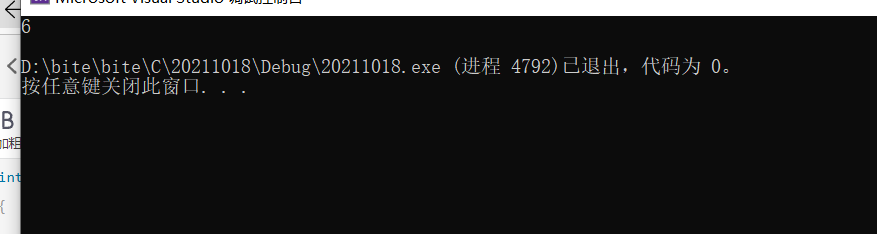## 7.3 递归与迭代

### 7.3.1 练习3：

``````#include<stdio.h>
//int Fac(int n)//非递归
//{
//	int i = 0;
//	int ret = 1;
//	for (i = 1; i <= n; i++)
//	{
//		ret *= i;
//	}
//	return ret;
//}

int Fac(int n)//递归
{
if (n <= 1)
return 1;
else
return n * Fac(n - 1);
}

int main()
{
//求阶乘
int n = 0;
scanf("%%%%d", &n);
int ret = Fac(n);
printf("%%%%d", ret);

return 0;
}
```12345678910111213141516171819202122232425262728293031```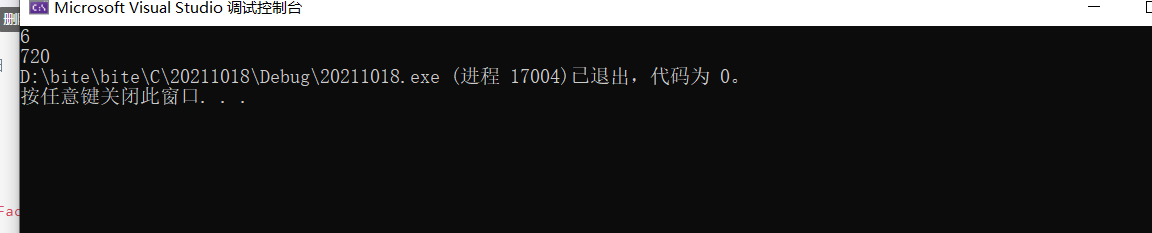### 7.3.2 练习4：

``````求第n个斐波那契数。（不考虑溢出）

//求第n个斐波那契数。（不考虑溢出）
//1 1 2 3 5 8 13 21 34 55
//前两个数字之和为第三个数字
//Fib(int n)
//n<=2, 1
//n>2,  Fib(n-1)+Fib(n-2)

//递归
#include<stdio.h>
int Fib(int n)
{
if (n <= 2)
return 1;
else
return Fib(n - 1) + Fib(n - 2);
}
int main()
{
int n = 0;
scanf("%%%%d", &n);
int ret = Fib(n);
printf("%%%%d ", ret);
}
```1234567891011121314151617181920212223242526```

``````#include<stdio.h>
int Fib(int n)
{
int a = 1;
int b = 1;
int c = 1;

while (n > 2)
{
c = a + b;
a = b;
b = c;
n--;
}
return c;
}
int main()
{
int n = 0;
scanf("%%%%d", &n);
int ret = Fib(n);
printf("%%%%d", ret);
return 0;
}
```123456789101112131415161718192021222324```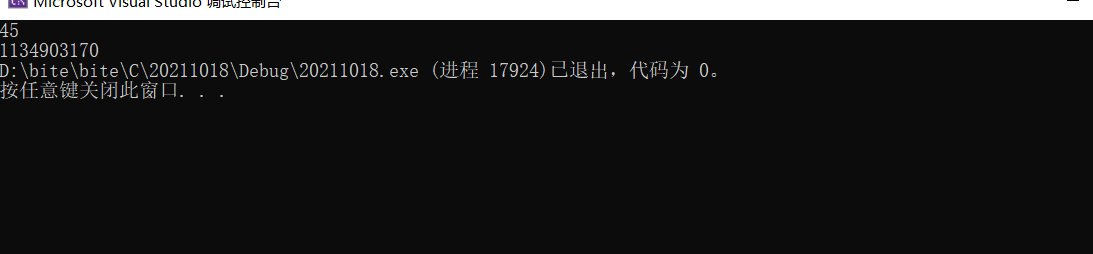1. 许多问题是以递归的形式进行解释的，这只是因为它比非递归的形式更为清晰。
2. 但是这些问题的迭代实现往往比递归实现效率更高，虽然代码的可读性稍微差些。
3. 当一个问题相当复杂，难以用迭代实现时，此时递归实现的简洁性便可以补偿它所带来的运行时开
销。

## 7.4函数递归的几个经典题目：汉诺塔问题、青蛙跳台阶问题

1. 汉诺塔问题
汉诺塔问题是一个经典的问题。汉诺塔（Hanoi Tower），又称河内塔，源于印度一个古老传说。大梵天创造世界的时候做了三根金刚石柱子，在一根柱子上从下往上按照大小顺序摞着64片黄金圆盘。大梵天命令婆罗门把圆盘从下面开始按大小顺序重新摆放在另一根柱子上。并且规定，任何时候，在小圆盘上都不能放大圆盘，且在三根柱子之间一次只能移动一个圆盘。问应该如何操作？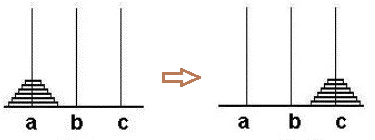``````//汉诺塔问题
//假设总共需要移动n个盘子
//1.将A柱上的n - 1个盘子借助C柱移向B柱
//2.将A柱上仅剩的最后一个盘子移向C柱
//3.将B柱上的n - 1个盘子借助A柱移向C柱
#include<stdio.h>
void move(int x, int y)
{
printf("%%%%c->%%%%c
", x, y);
}
void hanoi(int n, char a, char b, char c)
{
if (n == 1)
{
move(a, c);
}
else
{
hanoi(n - 1, a, c, b);//将A座上的n-1个盘子借助C座移向B座
move(a, c);//将A座上最后一个盘子移向C座
hanoi(n - 1, b, a, c);//将B座上的n-1个盘子借助A座移向C座
}
}
//move中的实参与hanoi函数中的形参相对应，而hanoi函数中形参a，b，c所对应的值也是在有规律的变化
int main()
{
int n = 0;
scanf("%%%%d", &n);
hanoi(n, 'A', 'B', 'C');
return 0;
}
```12345678910111213141516171819202122232425262728293031```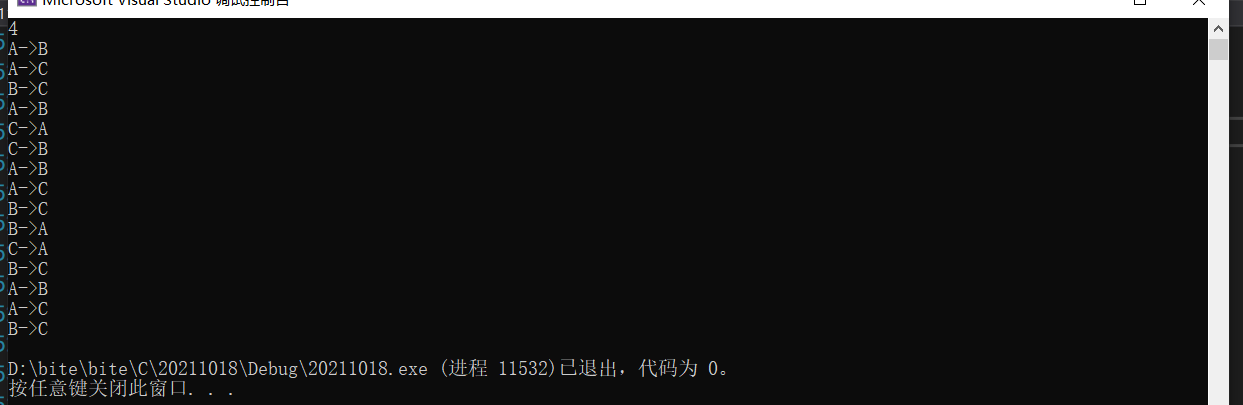2. 青蛙跳台阶问题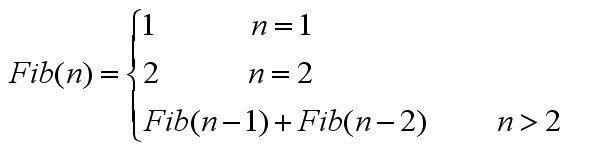``````#include<stdio.h>
int Fib(int n)
{
if (n <= 0)
{
printf("error");
return -1;
}

if (1 == n)
{
return 1;
}
else if (2 == n)
{
return 2;
}
else
{
return Fib(n - 1) + Fib(n - 2);
}
}

int main()
{
int n = 0;
scanf("%%%%d", &n);
int ret = Fib(n);
printf("一共有%%%%d 种跳发
", ret);
return 0;
}
```12345678910111213141516171819202122232425262728293031```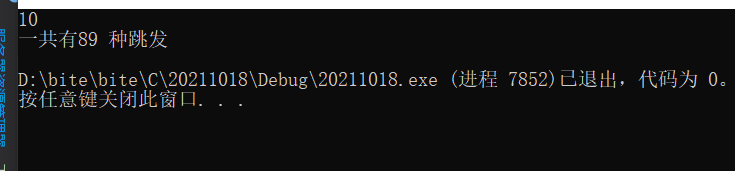## 7.5递归使用建议：

1.当解决一个问题递归和非递归都可以使用，且没有明显问题，那就可以使用递归
2.当问题用递归简单二非递归较难且递归没有明显问题，那就可以用递归
3.如果说用递归解决问题，写起来简单，但是有明显问题，就不能用递归，应该用非递归的方式解决问题